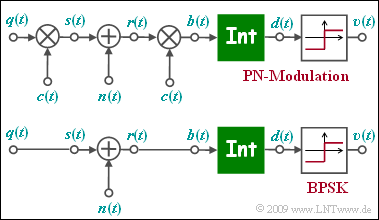# Exercise 3.7: PN ModulationEquivalent models for "PN modulation" and "BPSK"

The figure shows the equivalent block diagram of  "PN modulation"  or  "Direct Sequence Spread Spectrum", abbreviated  $\rm DS-SS$  in the equivalent low-pass range, where  $n(t)$  stands for AWGN noise. Below, the low-pass model of binary phase modulation  $\rm (BPSK)$  is sketched.

• The low-pass transmission signal  $s(t)$  is equal to the rectangular source signal  $q(t) ∈ \{+1, -1\}$  with rectangular duration  $T$  only for reasons of uniformity.
• The function of the integrator can be written as follows:
$$d (\nu T) = \frac{1}{T} \cdot \int_{(\nu -1 )T }^{\nu T} \hspace{-0.03cm} b (t )\hspace{0.1cm} {\rm d}t \hspace{0.05cm}.$$
• The two models differ in the multiplication by the  $±1$  spreading signal  $c(t)$  at the transmitter and the receiver.  Of the signal  $c(t)$  only the spread degree $J$  is known.
• The specification of the specific spreading sequence  $($"M sequence"  or  "Walsh function"$)$  is not important for the solution of this task.

It has to be examined whether the lower BPSK model can also be applied with  "PN modulation"  and whether the BPSK error probability

$$p_{\rm B} = {\rm Q} \left( \hspace{-0.05cm} \sqrt { {2 \cdot E_{\rm B}}/{N_{\rm 0}} } \hspace{0.05cm} \right )$$

is also valid for  "PN modulation", or how the given equation should be modified.

Notes:

### Questionnaire

1

Which values for the detection signal  $d(t)$  are possible with BPSK (without noise)?

 $d(\nu T)$  is Gaussian distributed. $d(\nu T)$  can take the values  $+1$,  $0$  and  $-1$ . Only the values  $d(\nu T) = +1$  and  $d(\nu T) = -1$  are possible.

2

Which values are possible with PN modulation in a noise-free case?

 $d(\nu T)$  is Gaussian distributed. $d(\nu T)$  can take the values  $+1$,  $0$  und  $-1$ . Only the values  $d(\nu T) = +1$  und  $d(\nu T) = -1$  are possible.

3

What modification must be done to the BPSK model so that it can also be used for PN modulation?

 The noise  $n(t)$  must be replaced by  $n\hspace{0.05cm}'(t) = n(t) \cdot c(t)$. The integration must now be done via  $J \cdot T$. The noise power must be reduced by the factor  $J$ .

4

The following applies   $10 \cdot {\rm lg}\ (E_{\rm B}/N_0) = 6 \ \rm dB$.  What error probability  $p_{\rm B}$  results with PN modulation?
Hint:   For BPSK, the result is  $p_{\rm B} \approx 2.3 \cdot 10^{-3}$.

 The larger  $J$  is selected, the smaller  $p_{\rm B}$  is. The larger  $J$  is selected, the larger  $p_{\rm B}$. It results always the value  $p_{\rm B} = 2.3 \cdot 10^{-3}$ results, independently from  $J$.

### Solution

#### Solution

(1)  Correct is the solution 3:

• This is an optimal receiver.
• Without noise, the signal  $b(t)$  is constantly equal to  $+1$  or  $-1$  within each bit.
• From the equation given for the integrator
$$d (\nu T) = \frac{1}{T} \cdot \hspace{-0.03cm} \int_{(\nu -1 )T }^{\nu T} \hspace{-0.3cm} b (t )\hspace{0.1cm} {\rm d}t$$
follows that  $d(\nu T)$  can only take the values  $±1$.

(2)  Correct is the solution 3

• In the noise-free case   ⇒   $n(t) = 0$, the twofold multiplication by  $c(t) ∈ \{+1, -1\}$   ⇒   $c(t)^{2} = 1$  can be omitted,
• so that the upper model is identical to the lower model.

(3)  The solution 1 is applicable:

• Since both models are identical in the noiseless case, only the noise signal has to be adjusted:  $n'(t) = n(t) \cdot c(t)$.
• The solutions 2 and 3 are not applicable:  Integration must still be done via  $T = J \cdot T_{c}$  $($not via  $J \cdot T)$  and PN modulation does not reduce the AWGN noise.

(4)  Correct is the solution 3:

• The equation valid for BPSK and AWGN channel is also applicable to PN modulation, independent of the spreading factor  $J$  and the specific spreading sequence:
$$p_{\rm B} = {\rm Q} \left( \hspace{-0.05cm} \sqrt { {2 \cdot E_{\rm B}}/{N_{\rm 0}} } \hspace{0.05cm} \right )$$
• For AWGN noise, the error probability is neither increased nor decreased by band spreading.